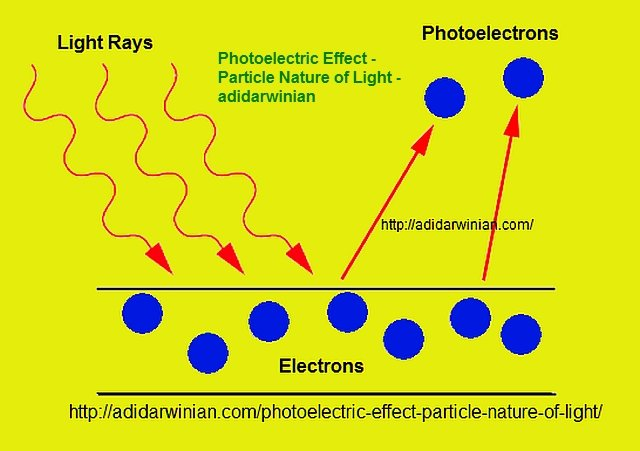# Photoelectric Effect – Particle Nature of Light

Modern physics fully accepts the concept of wave-particle duality in case of light and other electromagnetic radiation. The phenomena such as interference, diffraction, and polarization can only be explained when light is treated as a wave whereas the phenomena such as the photoelectric effect, line spectra, and the production and scattering of x rays demonstrate the particle nature of light. In this article, in order to show the particle nature of light, I have discussed the photoelectric effect along with the necessary equations.

The photoelectric effect is defined as a phenomenon in which the emission of electrons occurs when a beam of light strikes a metal or a cathode surface. For the emission of electrons to take place, the frequency of incident light is required to be greater than a certain minimum value. This value is known as the threshold frequency. The threshold frequency depends on the metal or material of the cathode. For most of the metals, threshold frequency is in the ultraviolet range (wavelengths between 200 nm to 300 nm). If the intensity of light (I) is increased while keeping the frequency same, more electrons are emitted per unit time. Thus, photocurrent is directly proportional to the intensity of light.

The photoelectric effect was correctly explained by the world-famous physicist, Albert Einstein, in the year 1905. It is interesting to know that Einstein was awarded the Nobel Prize in Physics 1921 for his work on photoelectric effect. Einstein figured out that a beam of light comprises of small packages of energy known as photons or quanta. The energy of a photon (E) is equal to the product of the Planck’s constant (h) and the frequency of a photon (f). Frequency (f) of photon = speed of light (c)/wavelength of photon (λ).

The energy of a photon is given by –

E = hf = hc/λ

Planck’s constant (h) = 6.6260755(40) x 10-34A photon, present in a beam of light, is absorbed by an electron present at a cathode or metal surface. The electron gets all of the photon’s energy or none at all. If the energy absorbed by the electron is greater than the work function for that particular metal or cathode surface, the electron may escape from the surface. Work function (ϕ) for a surface is defined as the minimum amount of energy required by an individual electron in order to escape from that particular surface.

Applying the law of conservation of energy, Einstein stated that the maximum kinetic energy (Kmax) for an electron emitted from a surface (a photoelectron) can be calculated by subtracting the work function (ϕ) from the energy gained from a photon (hf).

Kmax = 1/2mv2max = hf – ϕ

m = mass of electron

vmax = maximum velocity attained by an emitted electron

The maximum kinetic energy (Kmax) of a photoelectron can also be measured as eV0

Kmax = eV0

e is the magnitude of electron charge and is = 1.602 x 10-19 C

The stopping potential (V0) is the potential required to stop the emission of an electron from the surface of a cathode towards the anode.

Thus, putting Kmax = eV0 we get the equation of photoelectric effect:

eV0 = hf – ϕ

For a given cathode material, work function (ϕ) is constant. As e and h are also constant, V0 turns out to be a linear function of the frequency f. A graph of V0 as a function of frequency (f) is a straight line. The above equation of photoelectric effect also show that greater the work function of a metal or material, the higher the minimum frequency of light required to induce photoelectric effect, i.e., to cause emission of electrons (photoelectrons).